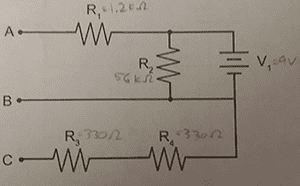# I thought circuits had to be closed

• Engineering
joel amos

## Homework Statement

I am supposed to determine the potential differences between the points. However, I thought circuits had to be closed...## Answers and Replies

Such a circuit would have to be closed in order for current to flow, but not for voltages to exist. If there is no current, then no voltage is dropped across each resistor.

Mentor
Circuits have to be closed for current to flow continuously, but potential difference does not depend upon a closed path or on continuous current flow. It is enough that there is some path between the points so that can do a "KVL walk" from one to the other, adding up the potential changes along the way.

joel amos
If there is no current, then no voltage is dropped across each resistor.
So how then do I calculate potential difference without current?

I'd usually use the formula V = I*R.

Mentor
So how then do I calculate potential difference without current?

I'd usually use the formula V = I*R.
Yes, and if I=0?

joel amos
Yes, and if I=0?
Well, then there would be no drop in voltage. Does this mean that the potential difference at all three points is the same?

EDIT: Well I guess there would be current through R2.

So then would the potential difference between A and B be 9 V, the difference between A and C be 9 V, and the difference C and B be 0 V?

Last edited:
Mentor
Well, then there would be no drop in voltage. Does this mean that the potential difference at all three points is the same?
Not quite. The battery will maintain a fixed potential difference regardless of current flow or not.
EDIT: Well I guess there would be current through R2.

So then would the potential difference between A and B be 9 V, the difference between A and C be 9 V, and the difference C and B be 0 V?
Yup.